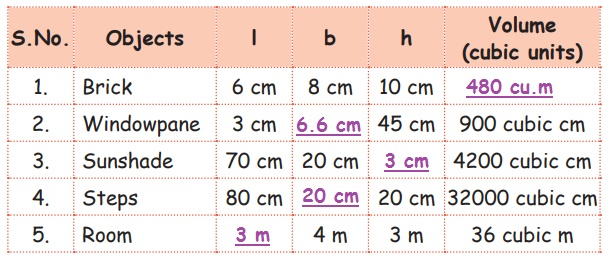Home | | Maths 5th Std | Exercise 3.2 (Finding volume using water, unit cubes)

# Exercise 3.2 (Finding volume using water, unit cubes)

Text Book Back Exercises Questions with Answers, Solution : 5th Maths : Term 3 Unit 3 : Measurements : Exercise 3.2 (Finding volume using water, unit cubes)

Exercise 3.2

1. Volume of regular solids such as cube and cuboid can be found by multiplying the dimensions. Complete the given table by finding the volume of the given objects.2. Complete the given table by finding the volume of the given objects.1. Volume = l × b × h

V =6 × 8 × 10

V = 480 cm3

2. Volume = l × b × h

900 = 3 × b × 45

b = 900 / [3 ×45] = 300/45

= 60/9 = 6.6

b = 6.6 cm

3. Volume = l × b × h

4200 =70 × 20 × h

h = 4200/ [70 × 20] = 42 / 14

h = 3cm

4. Volume = l × b × h

32000 = 80 × b × 20

b = 32000/ [80 × 20] = 320 / 16 = 36/12

b = 20cm

5. Volume = l × b × h

36 = 1 × 4 × 3

l = 36/ [4 ×3] = 36/12

l= 3 cm3. Find the number of bricks of dimension 20 cm × 5 cm × 10cm required to construct a wall of dimension 300 cm × 200 cm × 20 cm.

Step: 1

Length of brick = 20cm

Height of brick = 10cm

Volume of brick = l × b × h

= 20 × 5 ×10

Volumr of one brick = 1000 cu.cm

Step: 2

Length of wall = 300cm

Height of wall = 20cm

Volume of wall = l × b × h

= 300 × 200 × 20

= 1200000 cu.cm

Volume of wall = 12,00,000 cu.cm

Volume of one brick = 1000 cu.cm

No of brick required to construct a wall = 12,00,000 + 1000 = 1200

4. How many sack of dimension 15 cm × 45 cm × 90 cm filled with rice can be kept in a room of dimension 3 m × 18 m × 9 m.

Step: 1

Length of 1 sack = 15cm

Breadth of 1 sack = 45cm

Height of 1 sack = 90cm

Volume of 1 sack = l × b × h

= 15 × 45 × 90

Volume of one 1 sack = 60,750 cu.cm

Step: 2

Length of a room = 3m = 300cm

Breadth of a room = 18m = 1800cm

Height of a room = 9m = 900cm

Volume of a room = l × b × h

= 300 × 1800 × 900

= 486000000 cu.cm

Volume of room = 48,60,00,000 cu.cm

Volume of one sack = 60,750 cu.cm

No of sack = 486000000 ÷ 60750

= 8000

Answer: Total number of sack = 8000

Exercise 3.2

1. (i) 90 cu.cm  (ii) 3600 cu.cm  (iii) 3, 75, 000 cu.cm  (iv) 1000cu.cm (v) 1 cu.cm.

2. (i) 480 cu.cm (ii) 4cm (iii) 3cm (iv) 20 cm (vi) 3m

3. 1200 bricks

4. 8000 sacks

Tags : Measurements | Term 3 Chapter 3 | 5th Maths , 5th Maths : Term 3 Unit 3 : Measurements
Study Material, Lecturing Notes, Assignment, Reference, Wiki description explanation, brief detail
5th Maths : Term 3 Unit 3 : Measurements : Exercise 3.2 (Finding volume using water, unit cubes) | Measurements | Term 3 Chapter 3 | 5th Maths